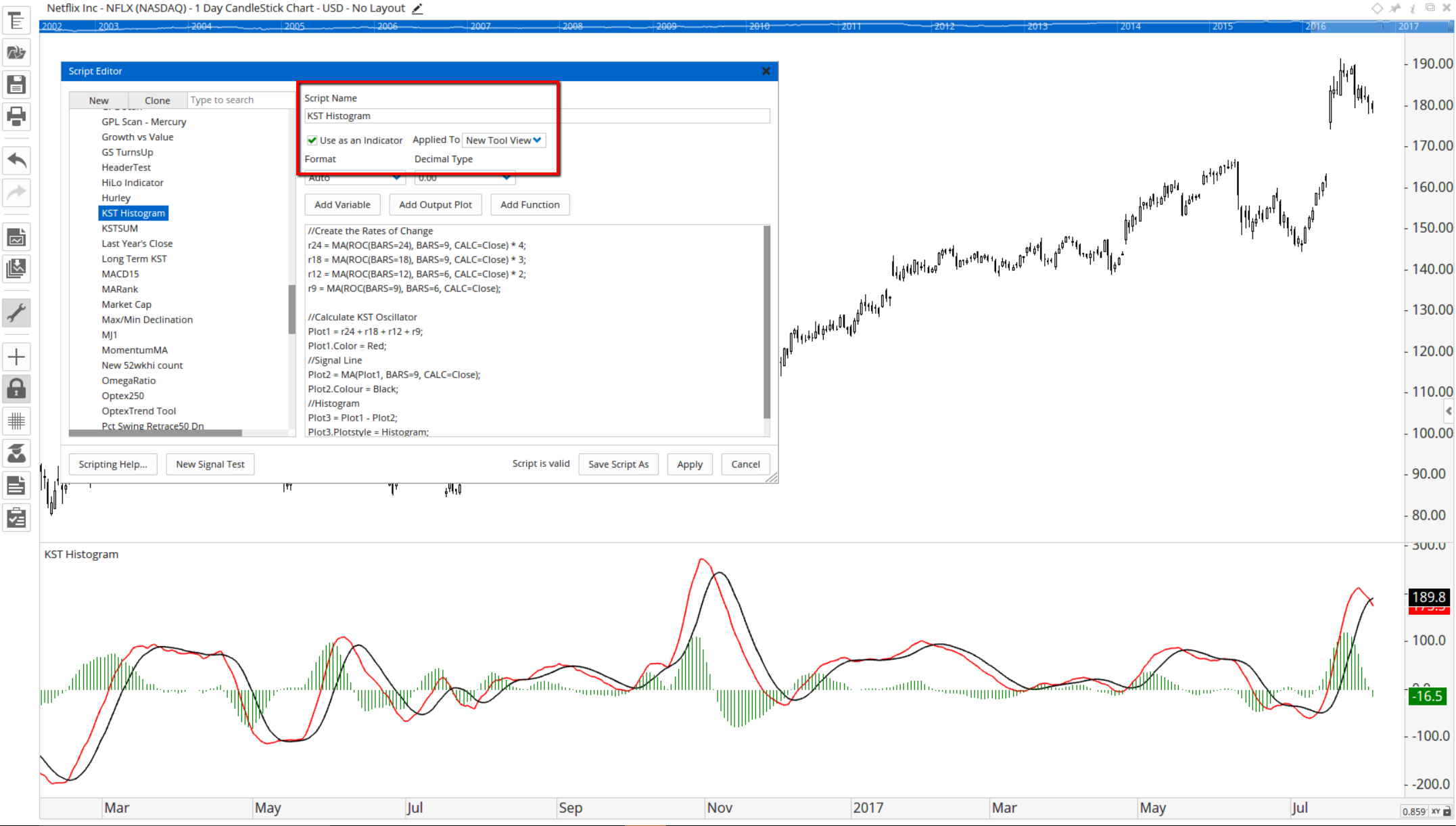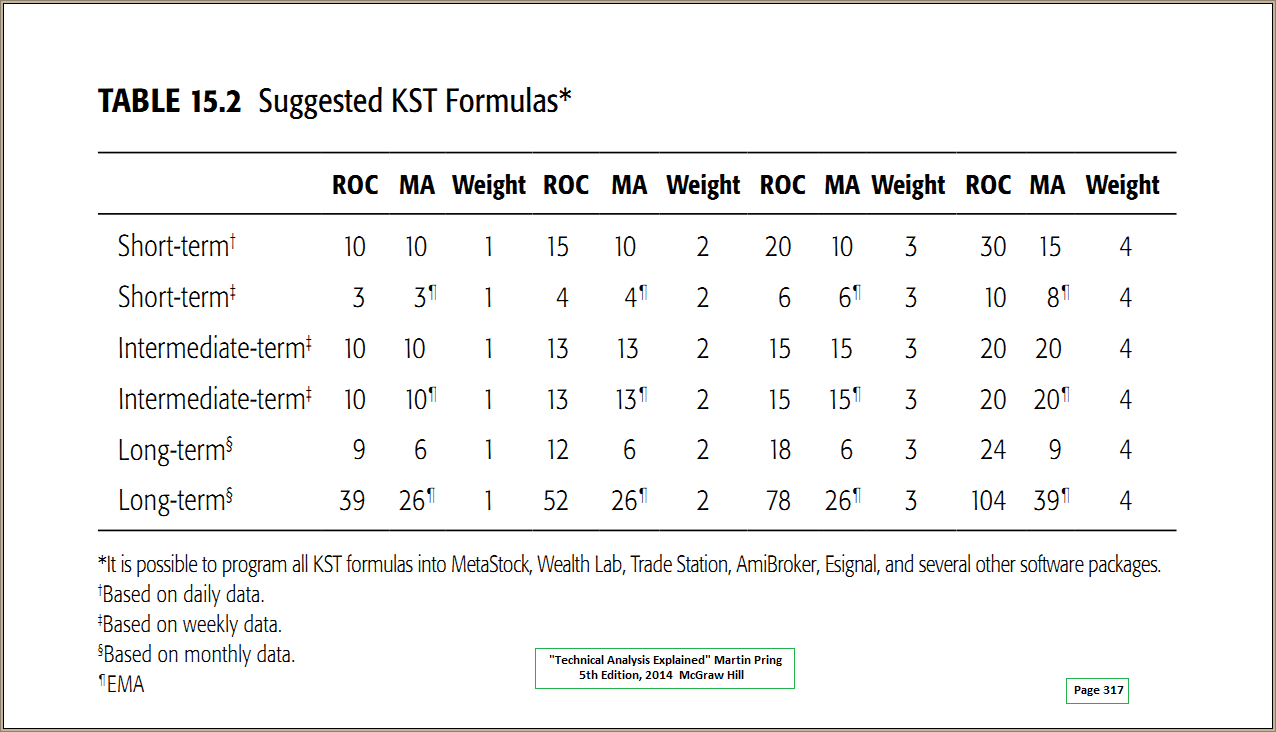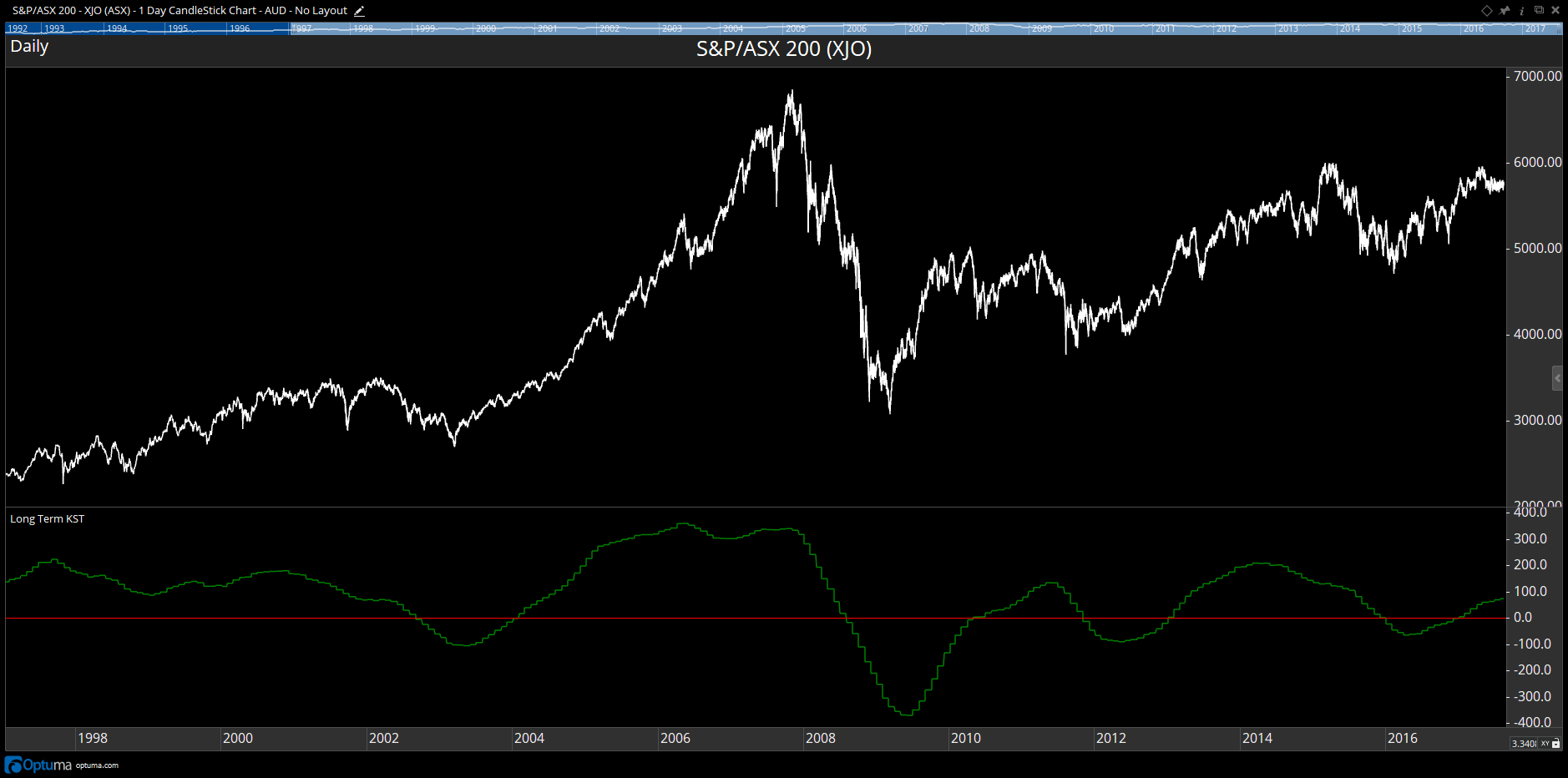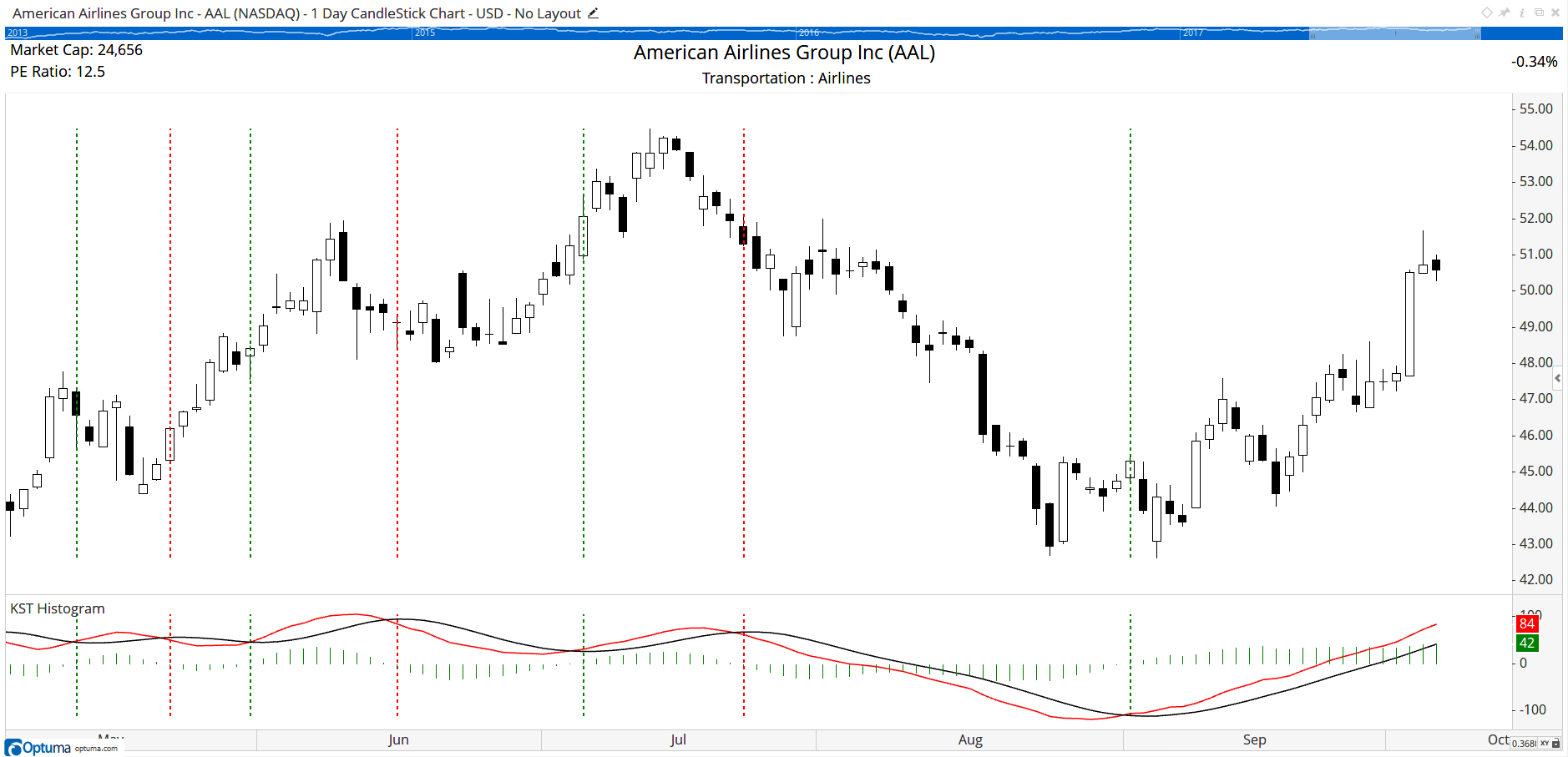Select Page

# Pring's KST Indicator

Optuma Forums Optuma Scripting Pring's KST Indicator

Viewing 7 posts - 1 through 7 (of 7 total)
• Author
Posts
• #38999

***UPDATE***

This tool is now included in the software, so no longer requires to be built using the scripting language.

***********************************

Developed by Martin Pring, Know Sure Thing (KST) is a momentum oscillator based on the smoothed rate-of-change for four different timeframes. In short, KST measures price momentum for four different price cycles. It can be used just like any momentum oscillator. Chartists can look for divergences, overbought/oversold readings, signal line crossovers and centerline crossovers.

//Create the averages of the Rates of Change
r24 = MA(ROC(BARS=24), BARS=9, CALC=Close) * 4;
r18 = MA(ROC(BARS=18), BARS=9, CALC=Close) * 3;
r12 = MA(ROC(BARS=12), BARS=6, CALC=Close) * 2;
r9 = MA(ROC(BARS=9), BARS=6, CALC=Close);

//Calculate KST Oscillator
Plot1 = r24 + r18 + r12 + r9;
Plot1.Color = Red;
//Signal Line
Plot2 = MA(Plot1, BARS=9, CALC=Close);
Plot2.Colour = Black;
//Histogram
Plot3 = Plot1-Plot2;
Plot3.Plotstyle = Histogram;

Save the script as an indicator in a new tool view and it will be added to your Toolbox:#39075

Hello Darren,

A few comments on the Pring’s KST Indicator.These parameters from Pring are in his book “Technical Analysis Explained”, 5th Edn, 2014, McGraw Hill; page 317.

The parameters you have used are for Weekly Data….the 5th set of parameters of the 6 offered.  May need to mention this to your viewers.

I coded the Daily Fast KST as below:

// ——-Know Sure Thing (KST) Indicator-FAST——-<br />// ——–Martin Pring—-Aug and Sept 1992———-<br />// ——-Signal…9 bars recommended…5 is better—<br />// ————STOCKS & COMMODITIES—————–<br />// ————————————————————–<br />RCMA1 = MA(ROC(10), BARS=10);<br />RCMA2 = MA(ROC(15), BARS=10);<br />RCMA3 = MA(ROC(20), BARS=10);<br />RCMA4 = MA(ROC(30), BARS=15);<br />// —calculate KST Oscillator and Signal—<br />KST = (RCMA1) + (RCMA2 * 2) + (RCMA3 * 3) + (RCMA4 * 4);<br />KSTsignal = MA(KST, BARS=5);<br />// —Plot KST Osc—<br />Plot1 = KST;<br />Plot1.Color = Red;<br />// —Plot KST Signal—<br />Plot2 = KSTsignal;<br />Plot2.Color = Blue;<br />// —Plot Histogram—<br />Plot3 = Plot1 – Plot2;<br />Plot3.PlotStyle = Histogram;

Works fine.   (..although yours is probably more elegantly coded).

Regards…..John

#39083

Darren,

My error….in the post above I said WEEKLY, should have said MONTHLY.

Apologies.

Regards….John

#39115

Thanks John. Here’s the monthly version:

RCMA1 = MA(ROC(MONTH(), BARS=9), BARS=6, CALC=Close);
RCMA2 = MA(ROC(MONTH(), BARS=12), BARS=6, CALC=Close) * 2;
RCMA3 = MA(ROC(MONTH(), BARS=18), BARS=6, CALC=Close) * 3;
RCMA4 = MA(ROC(MONTH(), BARS=24), BARS=9, CALC=Close) * 4;
RCMA1 + RCMA2 + RCMA3 + RCMA4#39251

Hi Darren,

When i copy past KST Histogram script in my optume error occur see in screen shot

#39323

Thanks Mohd. There was an issue with the minus sign when copied to the script editor, so please try copying and pasting the amended script above and see how you go.

#41659

To find when the KST crosses the signal line use the following in a Show Bar or scan formula.

Cross Above:

//Create the Rates of Change
r24 = MA(ROC(BARS=24), BARS=9, CALC=Close) * 4;
r18 = MA(ROC(BARS=18), BARS=9, CALC=Close) * 3;
r12 = MA(ROC(BARS=12), BARS=6, CALC=Close) * 2;
r9 = MA(ROC(BARS=9), BARS=6, CALC=Close);

KST1 = r24 + r18 + r12 + r9;
//Signal Line
KSTMA = MA(KST1, BARS=9, CALC=Close);
KST1 CrossesAbove KSTMA

Cross Below:

//Create the Rates of Change
r24 = MA(ROC(BARS=24), BARS=9, CALC=Close) * 4;
r18 = MA(ROC(BARS=18), BARS=9, CALC=Close) * 3;
r12 = MA(ROC(BARS=12), BARS=6, CALC=Close) * 2;
r9 = MA(ROC(BARS=9), BARS=6, CALC=Close);
KST1 = r24 + r18 + r12 + r9;
//Signal Line
KSTMA = MA(KST1, BARS=9, CALC=Close);
KST1 CrossesBelow KSTMA

In this example the Show Bar has been set to Lines instead of Arrows:Viewing 7 posts - 1 through 7 (of 7 total)
• You must be logged in to reply to this topic.